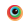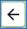• Official websites use .mass.gov

A .mass.gov website belongs to an official government organization in Massachusetts.

• Secure websites use HTTPS certificate

A lock icon ( ) or https:// means you’ve safely connected to the official website. Share sensitive information only on official, secure websites.

# QAG Info detail with landing page features

This is a test page used to verify that features are still working

This is a test page.

## section to show image in rich text

Here is some text. Here is some text. Here is some text. Here is some text. Here is some text. Here is some text. Here is some text. Here is some text. Here is some text. Here is some text. Here is some text. Here is some text. Here is some text. Here is some text. Here is some text. Here is some text. Here is some text. Here is some text. Here is some text. Here is some text. Here is some text. Here is some text. Here is some text. Here is some text. Here is some text. Here is some text. Here is some text. Here is some text. Here is some text. Here is some text. Here is some text. Here is some text. Here is some text. Here is some text. Here is some text. Here is some text. Here is some text. Here is some text. Here is some text. Here is some text. Here is some text. Here is some text.  The following image is full width.Here is some text. Here is some text. Here is some text. Here is some text. Here is some text. Here is some text. Here is some text. Here is some text. Here is some text. Here is some text. Here is some text. Here is some text. Here is some text. Here is some text. Here is some text. Here is some text. Here is some text. Here is some text. Here is some text. Here is some text. Here is some text. Here is some text. Here is some text. Here is some text. Here is some text. Here is some text. Here is some text. Here is some text. Here is some text. Here is some text. Here is some text. Here is some text. Here is some text. Here is some text. Here is some text. Here is some text. Here is some text. Here is some text. Here is some text. Here is some text. Here is some text. Here is some text. The following image is HALF width.Here is some text. Here is some text. Here is some text. Here is some text. Here is some text. Here is some text. Here is some text. Here is some text. Here is some text. Here is some text. Here is some text. Here is some text. Here is some text. Here is some text. Here is some text. Here is some text. Here is some text. Here is some text. Here is some text. Here is some text. Here is some text. Here is some text. Here is some text. Here is some text. Here is some text. Here is some text.Here is some text. Here is some text. Here is some text. Here is some text. Here is some text. Here is some text. Here is some text. Here is some text. Here is some text. Here is some text. Here is some text. Here is some text. Here is some text. Here is some text. Here is some text. Here is some text.

## Contact

#### Phone

Main office (617) 626-4400

Open Monday through Friday 8:30 a.m. - 4:30 p.m.

CommonHelp Service Desk (866) 888-2808

You can also email CommonHelpServiceDesk@mass.gov

#### Fax

Support requests 617-111-1111

Please submit requests by FAX only as a last resort.